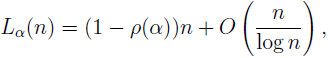# A note on the density of the Greatest Prime Factor

Rafael Jakimczuk
Notes on Number Theory and Discrete Mathematics, ISSN 1310-5132
Volume 19, 2013, Number 1, Pages 55—58

## Details

### Authors and affiliations

Rafael JakimczukDivisión Matemática, Universidad Nacional de Luján
Buenos Aires, Argentina

### Abstract

Let P(n) be the greatest prime factor of a positive integer n ≥ 2. Let L (n) be the number of 2 ≤ kn such that P(k) > kα, where 0 < α < 1. We prove the following asymptotic formulawhere ρ(α) is the Dickman’s function.

### Keywords

• Greatest prime factor
• Distribution

• 11A99
• 11B99

### References

1. Kemeny, J. Largest prime factor, J. Pure Appl. Algebra, Vol. 89, 1993, 181–186.
2. LeVeque, W. J. Topics in Number Theory, Addison-Wesley, 1958.
3. Ramaswami, R. On the number of positive integers less than x and free of prime divisors greater than xc, Bull. Amer. Math. Soc., Vol. 55, 1949, 1122–1127.

## Cite this paper

APA

Jakimczuk, R. (2013). A note on the density of the Greatest Prime Factor. Notes on Number Theory and Discrete Mathematics, 19(1), 55-58.

Chicago

Jakimczuk, Rafael. “A Note on the Density of the Greatest Prime Factor.” Notes on Number Theory and Discrete Mathematics 19, no. 1 (2013): 55-58.

MLA

Jakimczuk, Rafael. “A Note on the Density of the Greatest Prime Factor.” Notes on Number Theory and Discrete Mathematics 19.1 (2013): 55-58. Print.